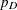The HPFMM Procedure

Default Output for Bayes Estimation

Bayes Information

This table provides basic information about the sampling algorithm. The HPFMM procedure uses either a conjugate sampler or a Metropolis-Hastings sampling algorithm based on Gamerman (1997). The table reveals, for example, how many model parameters are sampled, how many parameters associated with mixing probabilities are sampled, and how many threads are used to perform multithreaded analysis.

Prior Distributions

The "Prior Distributions" table lists for each sampled parameter the prior distribution and its parameters. The mean and variance (if they exist) for those values of the parameters are also displayed, along with the initial value for the parameter in the Markov chain. The Component column in this table identifies the mixture component to which a particular parameter belongs. You can control how the HPFMM procedure determines initial values with the INITIAL= option in the BAYES statement.

Bayesian Fit Statistics

The "Bayesian Fit Statistics" table shows three measures based on the posterior sample. The "Average -2 Log Likelihood" is derived from the average mixture log-likelihood for the data, where the average is taken over the posterior sample. The deviance information criterion (DIC) is a Bayesian measure of model fit and the effective number of parameters () is a penalization term used in the computation of the DIC. See the section Summary Statistics in SAS/STAT 14.1 User's Guide in Chapter 7: Introduction to Bayesian Analysis Procedures in SAS/STAT 14.1 User's Guide, for a detailed discussion of the DIC and.

Posterior Summaries

The arithmetic mean, standard deviation, and percentiles of the posterior distribution of the parameter estimates are displayed in the "Posterior Summaries" table. By default, the HPFMM procedure computes the 25th, 50th (median), and 75th percentiles of the sampling distribution. You can modify the percentiles through suboptions of the STATISTICS option in the BAYES statement. If a parameter corresponds to a singularity in the design and was removed from sampling for that purpose, it is also displayed in the table of posterior summaries (and in other tables that relate to output from the BAYES statement). The posterior sample size for such a parameter is shown as N = 0.

Posterior Intervals

The table of "Posterior Intervals" displays equal-tail intervals and intervals of highest posterior density for each parameter. By default, intervals are computed for an-level of 0.05, which corresponds to 95% intervals. You can modify this confidence level by providing one or morevalues in the ALPHA= suboption of the STATISTICS option in the BAYES statement. The computation of these intervals is detailed in section Summary Statistics in SAS/STAT 14.1 User's Guide in Chapter 7: Introduction to Bayesian Analysis Procedures in SAS/STAT 14.1 User's Guide.

Posterior Autocorrelations

Autocorrelations for the posterior estimates are computed by default for autocorrelation lags 1, 5, 10, and 50, provided that a sufficient number of posterior samples is available. See the section Assessing Markov Chain Convergence in SAS/STAT 14.1 User's Guide in Chapter 7: Introduction to Bayesian Analysis Procedures in SAS/STAT 14.1 User's Guide, for the computation of posterior autocorrelations and their utility in diagnosing convergence of Markov chains. You can modify the list of lags for which posterior autocorrelations are calculated with the AUTOCORR suboption of the DIAGNOSTICS= option in the BAYES statement.